# Calculate the Gradients of a Line

In this worksheet, students work out the gradient of a line on coordinate axes.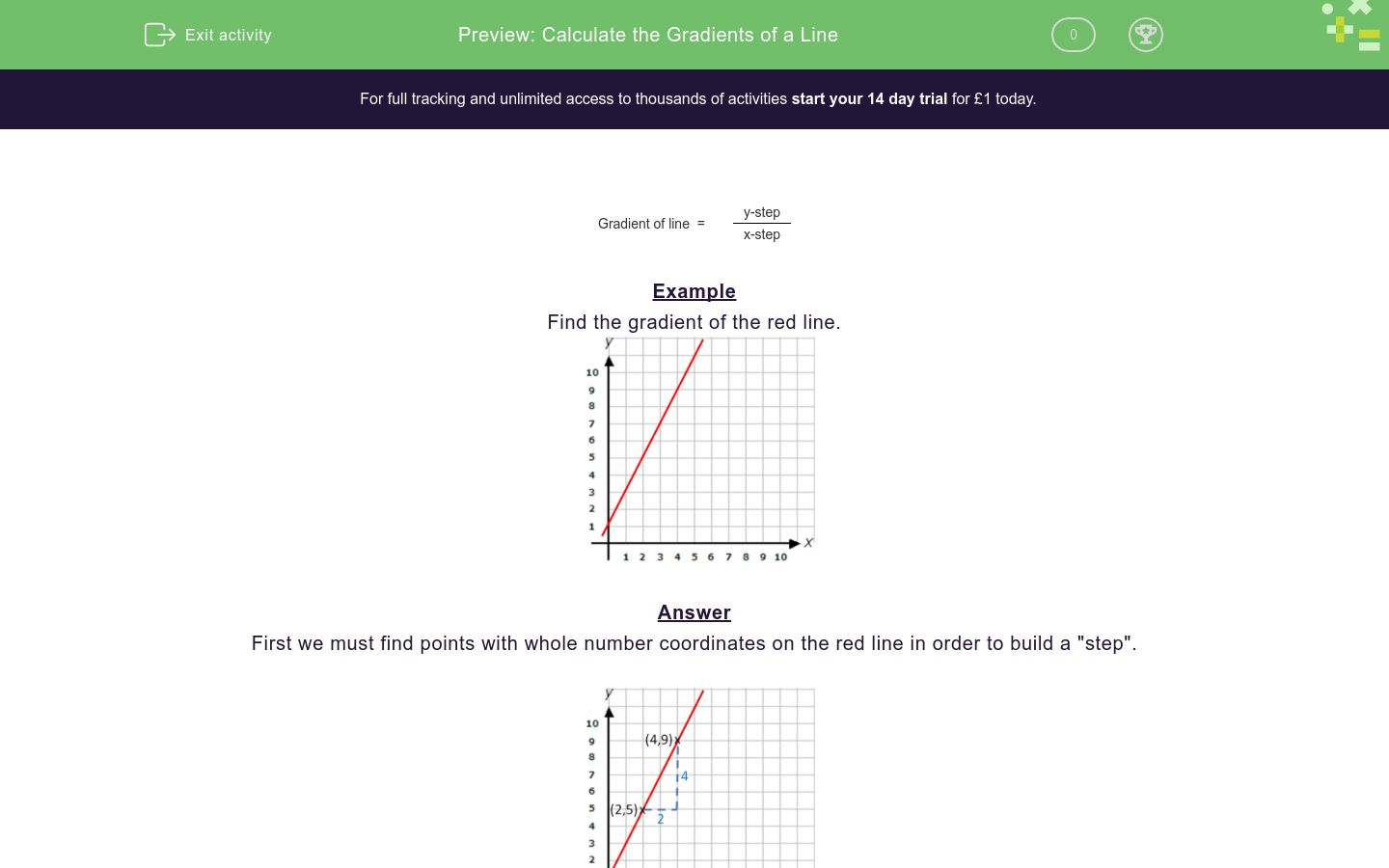Key stage:  KS 3

Curriculum topic:   Algebra

Curriculum subtopic:   Use Linear Equations (Two Variables) and Gradients

Difficulty level:### QUESTION 1 of 10

 Gradient of line  = y-step x-step

Example

Find the gradient of the red line.First we must find points with whole number coordinates on the red line in order to build a "step".The vertical part of the step (y-step) = 4

The horizontal part of the step (x-step) = 2

 Gradient of line  = y-step = 4 =  2 x-step 2

Find the gradient of the red line.

(If it is not a whole number, write it as a fraction in the form a/b)Find the gradient of the red line.

(If it is not a whole number, write it as a fraction in the form a/b)Find the gradient of the red line.

(If it is not a whole number, write it as a fraction in the form a/b)Find the gradient of the red line.

(If it is not a whole number, write it as a fraction in the form a/b)Find the gradient of the red line.

(If it is not a whole number, write it as a fraction in the form a/b)Find the gradient of the red line.

(If it is not a whole number, write it as a fraction in the form a/b)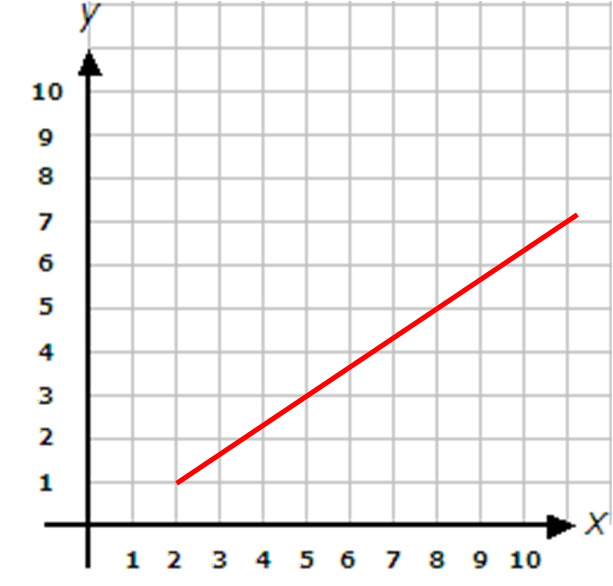Find the gradient of the red line.

(If it is not a whole number, write it as a fraction in the form a/b)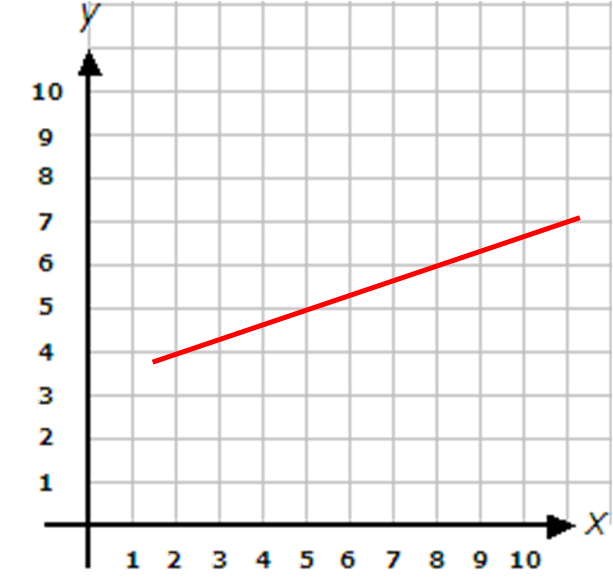Find the gradient of the red line.

(If it is not a whole number, write it as a fraction in the form a/b)Find the gradient of the red line.

(If it is not a whole number, write it as a fraction in the form a/b)Find the gradient of the red line.

(If it is not a whole number, write it as a fraction in the form a/b)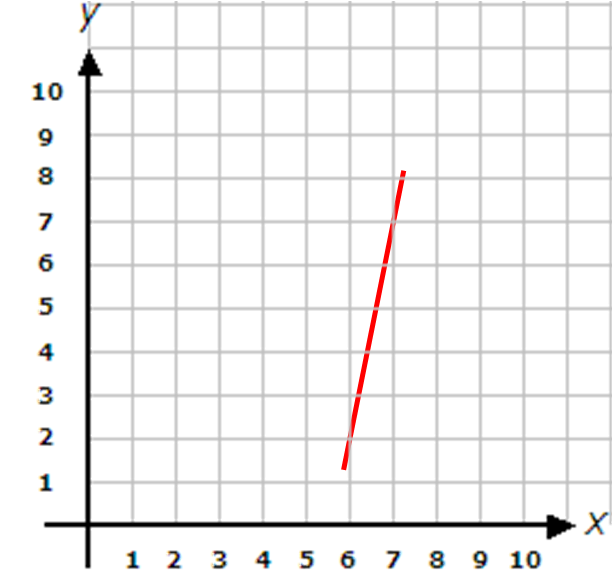• Question 1

Find the gradient of the red line.

(If it is not a whole number, write it as a fraction in the form a/b)2
EDDIE SAYSGradient of line  = y-step = 6 =  2 x-step 3
• Question 2

Find the gradient of the red line.

(If it is not a whole number, write it as a fraction in the form a/b)1
EDDIE SAYSGradient of line = y-step = 4 = 1 x-step 4
• Question 3

Find the gradient of the red line.

(If it is not a whole number, write it as a fraction in the form a/b)2/3
EDDIE SAYSGradient of line = y-step = 4 =2/3 x-step 6
• Question 4

Find the gradient of the red line.

(If it is not a whole number, write it as a fraction in the form a/b)5/2
EDDIE SAYSGradient of line = y-step = 5 =5/2 x-step 2
• Question 5

Find the gradient of the red line.

(If it is not a whole number, write it as a fraction in the form a/b)1/3
EDDIE SAYSGradient of line = y-step = 2 = 1/3 x-step 6
• Question 6

Find the gradient of the red line.

(If it is not a whole number, write it as a fraction in the form a/b)2/3
EDDIE SAYSGradient of line = y-step = 2 = 2/3 x-step 3
• Question 7

Find the gradient of the red line.

(If it is not a whole number, write it as a fraction in the form a/b)1/3
EDDIE SAYS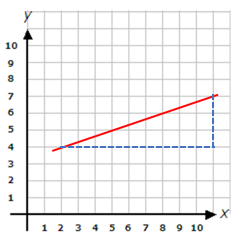Gradient of line = y-step = 3 = 1/3 x-step 9
• Question 8

Find the gradient of the red line.

(If it is not a whole number, write it as a fraction in the form a/b)1/2
EDDIE SAYSGradient of line = y-step = 2 = 1/2 x-step 4
• Question 9

Find the gradient of the red line.

(If it is not a whole number, write it as a fraction in the form a/b)1/4
EDDIE SAYS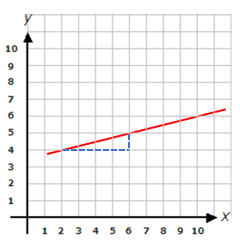Gradient of line = y-step = 1 = 1/4 x-step 4
• Question 10

Find the gradient of the red line.

(If it is not a whole number, write it as a fraction in the form a/b)5
EDDIE SAYS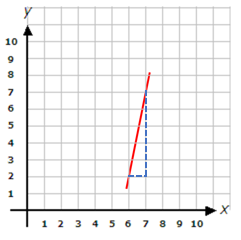Gradient of line = y-step = 5 = 5 x-step 1
---- OR ----

Sign up for a £1 trial so you can track and measure your child's progress on this activity.

### What is EdPlace?

We're your National Curriculum aligned online education content provider helping each child succeed in English, maths and science from year 1 to GCSE. With an EdPlace account you’ll be able to track and measure progress, helping each child achieve their best. We build confidence and attainment by personalising each child’s learning at a level that suits them.

Get started